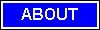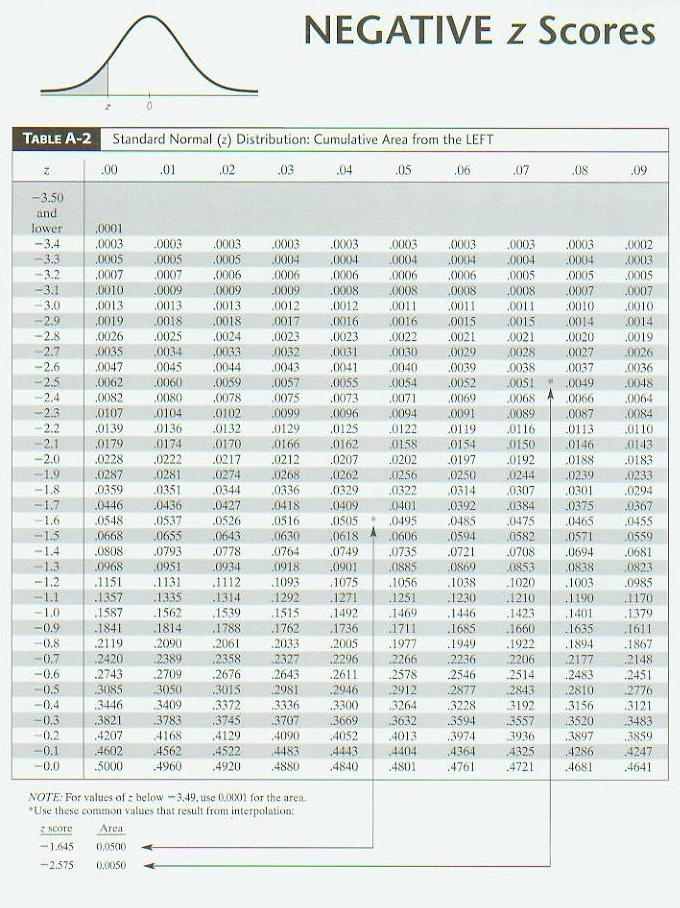M A T H   T U T O R I N G
ONE-ON-ONE
TEST PREPARATION917.392.7004    email: mathtutor255@yahoo.com

Statistics is the scientific application of mathematical principles to the collection, analysis, and presentation of numerical data. Statisticians contribute to scientific enquiry by applying their mathematical and statistical knowledge to the design of surveys and experiments; the collection, processing, and analysis of data; and the interpretation of the results. Statisticians may apply their knowledge of statistical methods to a variety of subject areas, such as biology, economics, engineering, medicine, public health, psychology, marketing, education, and sports. Randomness is studied using the mathematical discipline of probability theory. Probability is used in "mathematical statistics" (alternatively, "statistical theory") to study the sampling distributions of sample statistics and, more generally, the properties of statistical procedures. The use of any statistical method is valid when the system or population under consideration satisfies the assumptions of the method. The probability of event A is the number of ways event A can occur divided by the total number of possible outcomes. Let's take a look at a slight modification of the problem from the top of the page. Experiment 1: A spinner has 4 equal sectors colored yellow, blue, green and red. After spinning the spinner, what is the probability of landing on each color? Outcomes: The possible outcomes of this experiment are yellow, blue, green, and red. Probabilities: A single 6-sided die is rolled. What is the probability of each outcome? What is the probability of rolling an even number? of rolling an odd numbersitemap